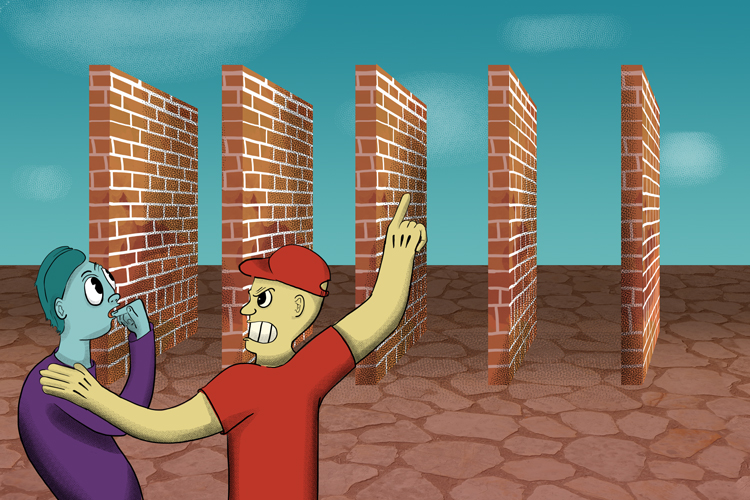# Multiplying column vectors

Multiplying vectors is just like any normal calculation but keep the top row separate from the bottom row. This is sometimes called scaling a vector. A scale has magnitude (size) only but in terms of scaling a vector you can think of scaling as a multiple.

Scalar = MultiplySCALE these walls MULTIPLE times to become world champion.

Example 1

Multiply vector ((3),(2))  by 3

((3),(2))times3=((3times3),(2times3))=((9),(6))

The vector ((3),(2)) multiplied by 3  is ((9),(6))

Example 2

Scale the vector ((3),(2))  by 3

((3),(2))times3=((3times3),(2times3))=((9),(6))

The vector ((3),(2)) scaled by 3  is ((9),(6))

Example 3

What is half of the vector ((2),(6))

((2),(6))times1/2=((2times1/2),(6times1/2))=((1),(3))

Half of the vector ((2),(6))=((1),(3))

Example 4

What is half of  the vector ((-4),(6))

((-4),(6))times1/2=((-4times1/2),(6times1/2))=((-2),(3))

Half of the vector ((-4),(6))=((-2),(3))